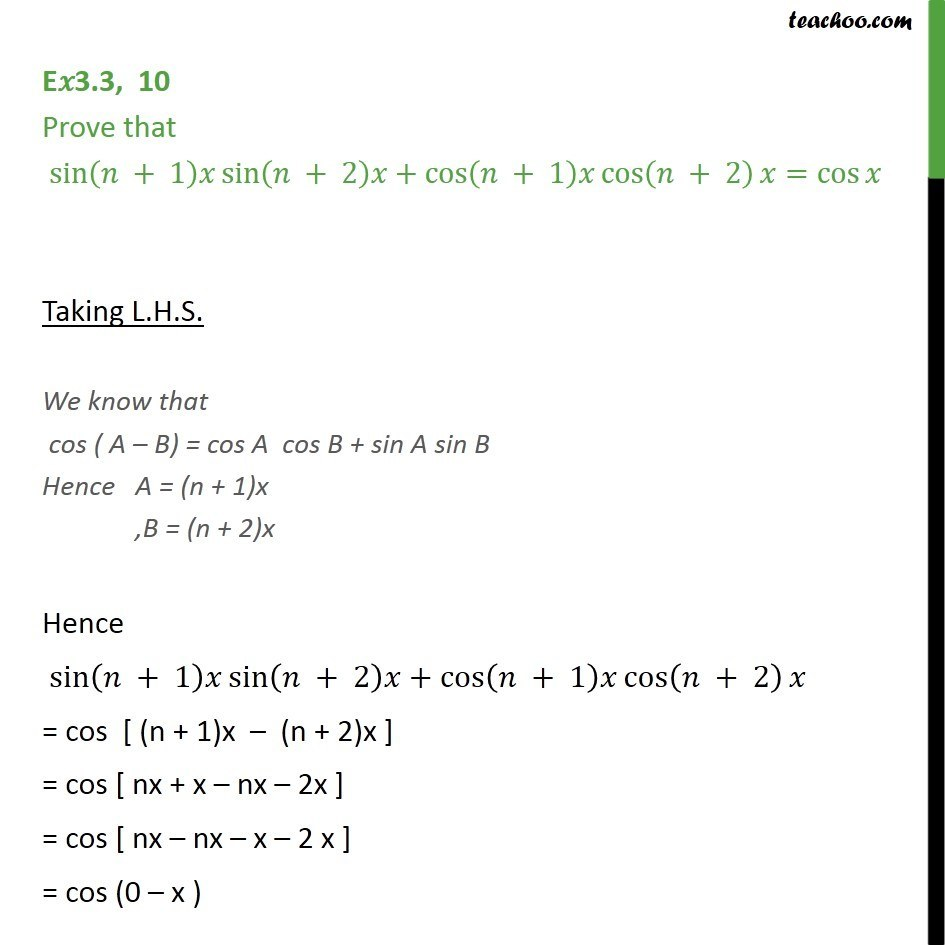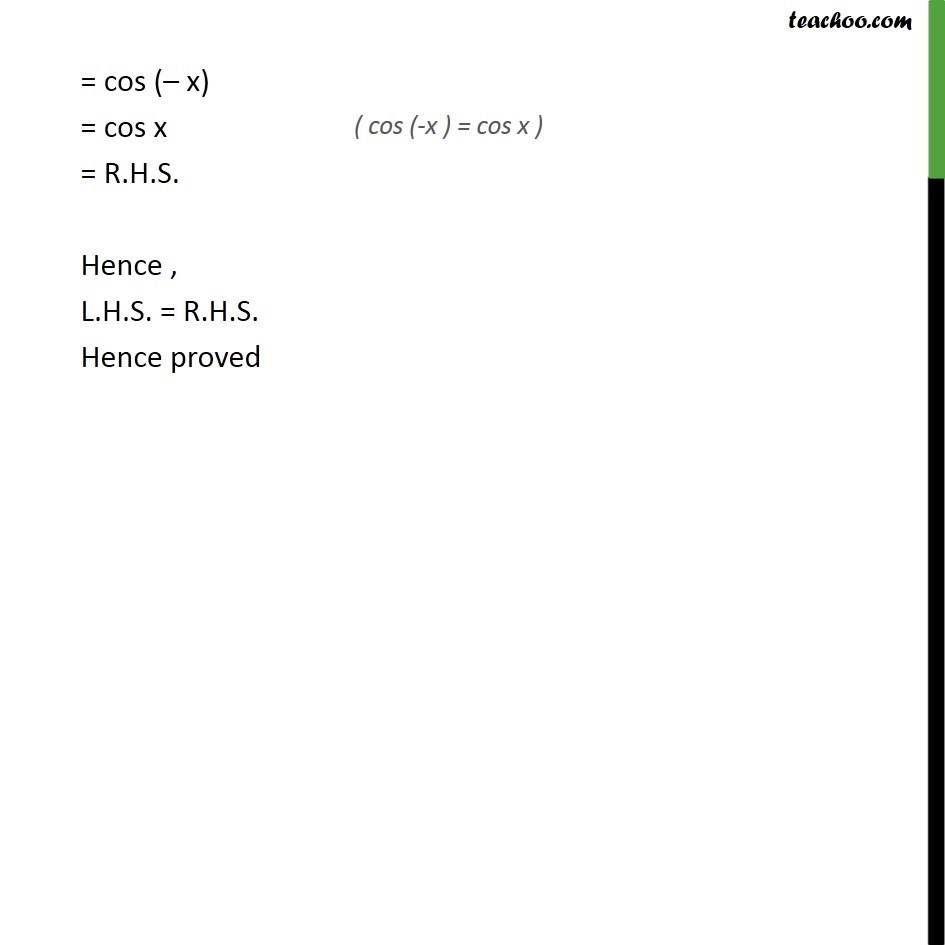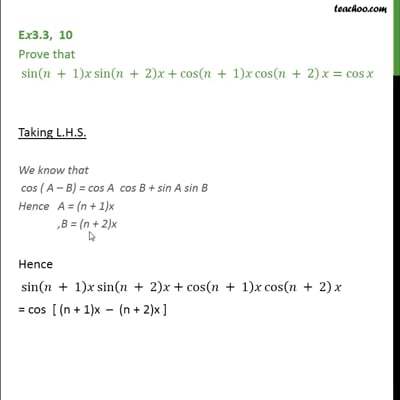(x + y) formula

Chapter 3 Class 11 Trigonometric Functions
Concept wiseThis video is only available for Teachoo black users

Introducing your new favourite teacher - Teachoo Black, at only ₹83 per month

### Transcript

E 3.3, 10 Prove that sin ( + 1) sin ( + 2) +cos ( + 1) cos ( + 2) =cos Taking L.H.S. We know that cos ( A B) = cos A cos B + sin A sin B Hence A = (n + 1)x ,B = (n + 2)x Hence sin ( + 1) sin ( + 2) +cos ( + 1) cos ( + 2) = cos [ (n + 1)x (n + 2)x ] = cos [ nx + x nx 2x ] = cos [ nx nx x 2 x ] = cos (0 x ) = cos ( x) = cos x = R.H.S. Hence , L.H.S. = R.H.S. Hence proved